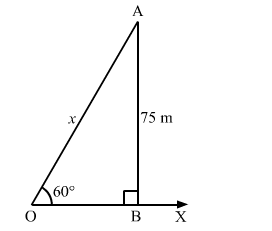# A kite is flying at a height of 75 m from the level ground,`
Question:

A kite is flying at a height of 75 m from the level ground, attached to a string inclined at a 60° to the horizontal. Find the length of the string assuming that there is no slack in it.

Solution:

Let $O X$ be the horizontal ground and $A$ be the position of the kite. Also, let $O$ be the position of the observer and $O A$ be the thread.

Now, draw $A B \perp O X$.

We have:

$\angle B O A=60^{\circ}, O A=75 \mathrm{~m}$ and $\angle O B A=90^{\circ}$

Height of the kite from the ground $=A B=75 \mathrm{~m}$

Length of the string, $O A=x \mathrm{~m}$In the right $\triangle O B A$, we have:

$\frac{A B}{O A}=\sin 60^{\circ}=\frac{\sqrt{3}}{2}$

$\Rightarrow \frac{75}{x}=\frac{\sqrt{3}}{2}$

$\Rightarrow x=\frac{75 \times 2}{\sqrt{3}}=\frac{150}{1.732}=86.6 \mathrm{~m}$

Hence, the length of the string is $86.6 \mathrm{~m}$.